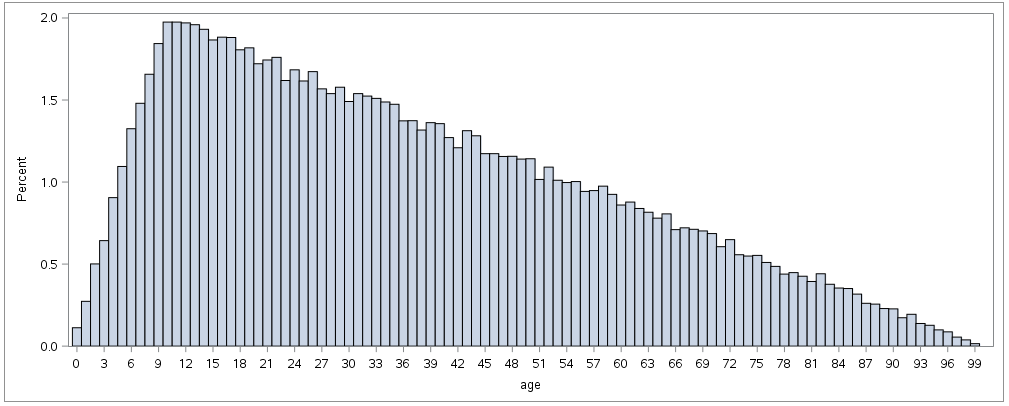## A simple histogram - how hard can it be?

I have been tearing my hair out with frustration for the last hour. I just want a histogram for my age distribution, in bins of 1 bar per age category (age in years, no decimals in the data), showing values 0, 5, 10 etc in the x-axis.

I am working from Enterprise Guide.

I cannot believe how many different procs there are to make histograms, each with different syntax. There is the

```proc univairate data=mydate;
histogram age;
run;```

or

```proc gchart data=mydata;
vbar age /discrete;
run;```

or

```PROC SGPLOT DATA = mydata;
HISTOGRAM age/binwidth=1 binstart=0;
TITLE "Age";
xaxis values=(0 to 100 by 1);
RUN; ```

etc.

The closest I have come is with the SGPLOT, but the first bar is halvway hidden behind the Y axis. Does anyone know how to make the first bar visible?Also, which command would you use to generate a simple histogram like this? Is there something like a best practice? Are some of these older procs getting faded out?

1 ACCEPTED SOLUTION

Accepted Solutions

## Re: A simple histogram - how hard can it be?

You're correct that there are many ways to create a histogram -- you touched only on a few of them!

I think the simplest method is the one you tried last: PROC SGPLOT.  Since you have 100 bins and the graph is only so wide, the algorithm to make everything fit might make your left-most extreme value seem very tight against the axis.  You can use the OFFSETMIN= option to give a little more space.  Try this:

``````data sample (keep=age);
do i = 1 to 100000;
age = abs ( floor ( rand('triangle',0.1) * 100 ) );
output;
end;
run;

ods graphics / width=1000px height=400px;
proc sgplot data=sample;
HISTOGRAM age / binwidth=1 binstart=0 ;
TITLE "Age";
xaxis values=(0 to 100 by 1) offsetmin=.01 offsetmax=.01 ;
RUN; ``````4 REPLIES 4

## Re: A simple histogram - how hard can it be?

You're correct that there are many ways to create a histogram -- you touched only on a few of them!

I think the simplest method is the one you tried last: PROC SGPLOT.  Since you have 100 bins and the graph is only so wide, the algorithm to make everything fit might make your left-most extreme value seem very tight against the axis.  You can use the OFFSETMIN= option to give a little more space.  Try this:

``````data sample (keep=age);
do i = 1 to 100000;
age = abs ( floor ( rand('triangle',0.1) * 100 ) );
output;
end;
run;

ods graphics / width=1000px height=400px;
proc sgplot data=sample;
HISTOGRAM age / binwidth=1 binstart=0 ;
TITLE "Age";
xaxis values=(0 to 100 by 1) offsetmin=.01 offsetmax=.01 ;
RUN; ``````## Re: A simple histogram - how hard can it be?

Thank you! Don't think I would have found that sollution on my own.

Is there a simple way to split this chart by say gender? So that I get a stacked histogram?

## Re: A simple histogram - how hard can it be?

Of course! Again, multple methods, but now it sounds like you're more interested in a VBAR with a grouping variable than a classic histogram of statistical distribution.

You could try PROC SGPANEL with a HISTOGRAM statement and PANELBY for gender (that would yield two histograms).  Or you could use PROC FREQ to calc the percentages into a data set, then use a step like:

``````proc sgplot data=freq_output;
vbar age / response=percent group=gender grouporder=data;
run;``````DanH_sas
SAS Super FREQ

## Re: A simple histogram - how hard can it be?

Building on Chris' example, here are three variations you do with your grouping variable

``````proc format;
value gender 1="Male"
2="Female"
;
run;

data sample (keep=age g);
do g = 1 to 2;
do i = 1 to 100000;
age = abs ( floor ( rand('triangle',0.1) * 100 ) );
output;
end;
end;
run;

ods graphics / width=1000px height=400px;
proc sgplot data=sample;
format g gender.;
by g;
HISTOGRAM age / binwidth=1 binstart=0 ;
TITLE "Age";
xaxis values=(0 to 100 by 1) offsetmin=.01 offsetmax=.01 ;
RUN;

proc sgplot data=sample;
format g gender.;
HISTOGRAM age / binwidth=1 binstart=0 group=g transparency=0.5;
TITLE "Age";
xaxis values=(0 to 100 by 1) offsetmin=.01 offsetmax=.01 ;
RUN;

proc sgpanel data=sample;
format g gender.;
panelby g / layout=rowlattice novarname;
HISTOGRAM age / binwidth=1 binstart=0 ;
TITLE "Age";
colaxis values=(0 to 100 by 1) offsetmin=.01 offsetmax=.01 ;
RUN;
``````
Discussion stats
• 4 replies
• 7753 views
• 4 likes
• 3 in conversation